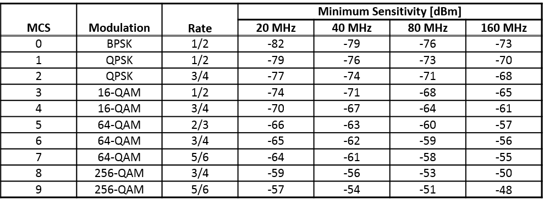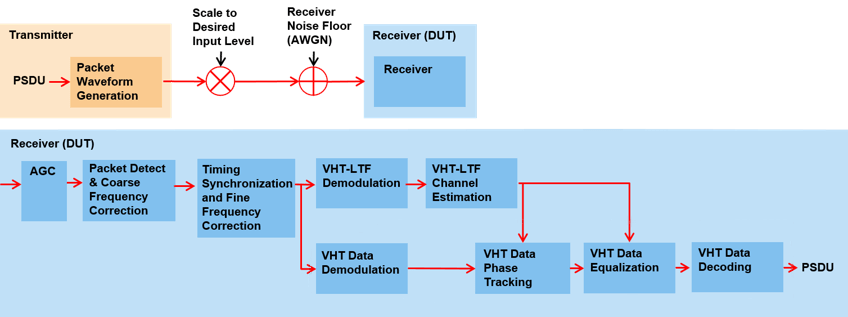Documentation

## 802.11ac Receiver Minimum Input Sensitivity Test

This example shows how to simulate a test to measure the receiver minimum input sensitivity as specified in Section 22.3.19.1 of the IEEE® 802.11ac™ standard [ 1 ].

### Introduction

The receiver minimum sensitivity test ensures a device under test (DUT) is able to receive data with a defined maximum packet error rate (PER) of 10% at a defined minimum signal power. The minimum signal power depends on the channel bandwidth and modulation and coding scheme (MCS) as specified in Table 22-25 of the IEEE 802.11ac standard [ 1 ]:When the test is performed with hardware, each input antenna port on the DUT is connected through a cable to a single output antenna port of a transmitter. The following transmission parameters are specified for the test waveform:

• The number of spatial streams is equal to the number of transmitting antenna ports

• PSDU length of 4096 bytes

• No space-time block coding (STBC)

• 800ns guard interval

• Binary convolutional coding

This example shows how the above test can be constructed with an end-to-end simulation using WLAN Toolbox™. In this example a receiver is stimulated with incoming VHT packets at a range of input levels below the minimum sensitivity level and the PER measured.

For each sensitivity level tested packets are generated and scaled to the desired signal level. White Gaussian noise is added to create a noise floor at the receiver. The noisy packets are then demodulated and the PSDUs recovered. The PSDUs are compared to those transmitted to determine the number of packet errors and hence the packet error rate. Automatic gain control (AGC), packet detection, timing synchronization, carrier frequency offset correction, noise estimation and phase tracking are performed by the receiver. The processing for each packet is summarized in the following diagram:### Test Parameters

The transmission configuration for the test is specified with a VHT configuration object. In this example the minimum sensitivity is measured for a 160 MHz transmission with 64-QAM rate 5/6 modulation and coding. The simulated DUT has 2 receive antennas. These parameters can be changed to test different configurations.

```cfgVHT = wlanVHTConfig; % Create VHT transmission configuration cfgVHT.ChannelBandwidth = 'CBW160'; % Bandwidth cfgVHT.MCS = 7; % 64-QAM, rate 5/6 NumReceiveAntennas = 2; % Number of receive antennas ```

The fixed transmission parameters required by the test are set below.

```cfgVHT.APEPLength = 4096; % Bytes cfgVHT.STBC = false; cfgVHT.NumTransmitAntennas = NumReceiveAntennas; cfgVHT.NumSpaceTimeStreams = NumReceiveAntennas; cfgVHT.SpatialMapping = 'Direct'; cfgVHT.GuardInterval = 'Long'; ```

### Simulation Parameters

In this example a receiver is stimulated with VHT packets at a range of input levels below the minimum input sensitivity level. The range of offsets tested is specified in the vector `testInputLevelOffsets`.

```testInputLevelOffsets = [-10 -9 -8 -7]; % dB ```

The number of packets tested at each sensitivity is controlled by two parameters:

1. `maxNumErrors` is the maximum number of packet errors simulated at each input level. When the number of packet errors reaches this limit, the simulation at this sensitivity level is complete.

2. `maxNumPackets` is the maximum number of packets simulated at each input level and limits the length of the simulation if the packet error limit is not reached.

The numbers chosen in this example will lead to a very short simulation. For meaningful results we recommend increasing the numbers.

```maxNumErrors = 20; maxNumPackets = 200; ```

### Signal Power Setup

The minimum sensitivity test specifies a maximum PER for a measured input level per receive antenna. In this simulation the receiver is stimulated with a test signal with a specified input level in dBm. The test signal is generated with the waveform generator, wlanWaveformGenerator. The output of the waveform generator is normalized internally such that the power for all antennas sums to 0 dBm. Therefore for this simulation the output of the waveform generator must be scaled to create the desired input level.

First the minimum sensitivity for the transmission configuration is determined from Table 22-25 of the IEEE 802.11ac standard [ 1 ].

```% Receiver minimum input level sensitivity for 20 MHz, Table 22-25. The % sensitivity increases by 3dB for double the bandwidth. rxMinSensitivityTable = [-82 -79 -77 -74 -70 -66 -65 -64 -59 -57]; % dBm % Get minimum input sensitivity given MCS and bandwidth fs = wlanSampleRate(cfgVHT); % Baseband sampling rate (Hz) B = floor(10*log10((fs/20e6))); % Scalar for bandwidth rxMinSensitivity = rxMinSensitivityTable(cfgVHT.MCS+1)+B; % dBm disp(['Minimum sensitivity for MCS' num2str(cfgVHT.MCS) ', ' ... num2str(fs/1e6) ' MHz: ' num2str(rxMinSensitivity,'%2.1f') ' dBm']) ```
```Minimum sensitivity for MCS7, 160 MHz: -55.0 dBm ```

In this example a range of input levels below the minimum level are tested. These power levels are defined by `testInputLevels`.

```testInputLevels = rxMinSensitivity+testInputLevelOffsets; % dBm ```

A voltage scalar, `A`, is calculated to scale the generated waveform for each test level. The power per receive antenna port is measured during the simulation to confirm the input signal level is correct.

```A = 10.^((testInputLevels-30)/20); % Voltage gain (attenuation) A = A*sqrt(cfgVHT.NumTransmitAntennas); % Account for generator scaling ```

### Noise Configuration

The noise floor of the receiver is simulated with thermal noise. The height of the noise floor determines the SNR at the receiver, as the input signal level is fixed for this test. The noise figure of the receiver determines the level of noise floor.

```NF = 6; % Noise figure (dB) T = 290; % Ambient temperature (K) BW = fs; % Bandwidth (Hz) k = 1.3806e-23; % Boltzmann constant (J/K) noiseFloor = 10*log10(k*T*BW)+NF; % dB disp(['Receiver noise floor: ' num2str(noiseFloor+30,'%2.1f') ' dBm']) ```
```Receiver noise floor: -85.9 dBm ```

An AWGN channel, comm.AWGNChannel, is used to add noise to the waveform.

```awgnChannel = comm.AWGNChannel('NoiseMethod','Variance', ... 'Variance',10^(noiseFloor/10)); ```

### Input Level Sensitivity Simulation

For each input level a number of packets are tested and the packet error rate calculated.

For each packet the following processing steps occur:

1. A PSDU is created and encoded to create a single packet waveform.

2. The waveform is scaled to create the desired input level in dBm.

3. The power of the received waveform is measured.

4. AWGN is added to the received waveform to create a noise floor.

5. The received waveform is passed through an automatic gain control to boost the signal prior to receiver processing.

6. The packet is detected.

7. Coarse carrier frequency offset is estimated and corrected.

8. Fine timing synchronization is established. The L-STF, L-LTF and L-SIG samples are provided for fine timing to allow for packet detection at the start or end of the L-STF.

9. Fine carrier frequency offset is estimated and corrected.

10. The VHT-LTF is extracted from the synchronized received waveform. The VHT-LTF is OFDM demodulated and channel estimation is performed.

11. The VHT Data field is extracted from the synchronized received waveform. The PSDU is recovered using the extracted field and the channel estimate.

```ind = wlanFieldIndices(cfgVHT); % For accessing fields within the packet chanBW = cfgVHT.ChannelBandwidth; rng(0); % Set random state for repeatability agc = comm.AGC; % Automatic gain control S = numel(testInputLevels); packetErrorRate = zeros(S,1); rxAntennaPower = zeros(S,1); for i=1:S disp(['Simulating ' num2str(testInputLevels(i),'%2.1f') ... ' dBm input level...']); % Loop to simulate multiple packets numPacketErrors = 0; measuredPower = zeros(maxNumPackets,1); % Average power per antenna numPkt = 1; % Index of packet transmitted while numPacketErrors<=maxNumErrors && numPkt<=maxNumPackets % Generate a packet waveform txPSDU = randi([0 1],cfgVHT.PSDULength*8,1); % PSDULength in bytes tx = wlanWaveformGenerator(txPSDU,cfgVHT); % Scale input signal to desired level rx = tx.*A(i); % Measure the average power at the antenna connector in Watts measuredPower(numPkt) = mean(mean(rx.*conj(rx))); % Add noise floor at receiver rx = awgnChannel(rx); % Pass each channel through AGC for ic = 1:size(rx,2) rx(:,ic) = agc(rx(:,ic)); reset(agc); end % Packet detect and determine coarse packet offset coarsePktOffset = wlanPacketDetect(rx,chanBW); if isempty(coarsePktOffset) % If empty no L-STF detected; packet error numPacketErrors = numPacketErrors+1; numPkt = numPkt+1; continue; % Go to next loop iteration end % Extract L-STF and perform coarse frequency offset correction lstf = rx(coarsePktOffset+(ind.LSTF(1):ind.LSTF(2)),:); coarseFreqOff = wlanCoarseCFOEstimate(lstf,chanBW); rx = helperFrequencyOffset(rx,fs,-coarseFreqOff); % Extract the non-HT fields and determine fine packet offset nonhtfields = rx(coarsePktOffset+(ind.LSTF(1):ind.LSIG(2)),:); finePktOffset = wlanSymbolTimingEstimate(nonhtfields,chanBW); % Determine final packet offset pktOffset = coarsePktOffset+finePktOffset; % if packet detected out of a reasonable range (>50 samples); % packet error if pktOffset>50 numPacketErrors = numPacketErrors+1; numPkt = numPkt+1; continue; % Go to next loop iteration end % Extract L-LTF and perform fine frequency offset correction lltf = rx(pktOffset+(ind.LLTF(1):ind.LLTF(2)),:); fineFreqOff = wlanFineCFOEstimate(lltf,chanBW); rx = helperFrequencyOffset(rx,fs,-fineFreqOff); % Extract VHT-LTF samples from the waveform, demodulate and perform % channel estimation vhtltf = rx(pktOffset+(ind.VHTLTF(1):ind.VHTLTF(2)),:); vhtltfDemod = wlanVHTLTFDemodulate(vhtltf,cfgVHT); chanEst = wlanVHTLTFChannelEstimate(vhtltfDemod,cfgVHT); % Get single stream channel estimate chanEstSSPilots = vhtSingleStreamChannelEstimate(vhtltfDemod,cfgVHT); % Extract VHT Data samples from the waveform vhtdata = rx(pktOffset+(ind.VHTData(1):ind.VHTData(2)),:); % Estimate the noise power in VHT data field nEstVHT = vhtNoiseEstimate(vhtdata,chanEstSSPilots,cfgVHT); % Recover the transmitted PSDU in VHT Data rxPSDU = wlanVHTDataRecover(vhtdata,chanEst,nEstVHT,cfgVHT); % Determine if any bits are in error, i.e. a packet error packetError = any(biterr(txPSDU,rxPSDU)); numPacketErrors = numPacketErrors+packetError; numPkt = numPkt+1; end % Calculate packet error rate (PER) at input level point packetErrorRate(i) = numPacketErrors/(numPkt-1); disp([' Completed after ' ... num2str(numPkt-1) ' packets, PER: ' ... num2str(packetErrorRate(i))]); % Calculate average input power per antenna rxAntennaPower(i) = 10*log10(mean(measuredPower(1:(numPkt-1))))+30; disp([' Measured antenna connector power: ' ... num2str(rxAntennaPower(i),'%2.1f') ' dBm']); end ```
```Simulating -65.0 dBm input level... Completed after 21 packets, PER: 1 Measured antenna connector power: -65.0 dBm Simulating -64.0 dBm input level... Completed after 26 packets, PER: 0.80769 Measured antenna connector power: -64.0 dBm Simulating -63.0 dBm input level... Completed after 130 packets, PER: 0.16154 Measured antenna connector power: -63.0 dBm Simulating -62.0 dBm input level... Completed after 200 packets, PER: 0.02 Measured antenna connector power: -62.0 dBm ```

### Analysis and Further Exploration

The PER for tested input signal levels is plotted with the maximum PER at minimum sensitivity.

```figure semilogy(rxAntennaPower,packetErrorRate,'o-') hold on semilogy(rxMinSensitivity,0.1,'rx') currentxlim = xlim(gca); xlim([currentxlim(1) currentxlim(2)+1]) grid on xlabel('Measured power per antenna connector (dBm)'); ylabel('PER'); legend('Simulated PER performance','Maximum PER at minimum sensitivity'); title(sprintf(['Minimum Input Sensitivity Test: MCS%d, %d MHz, ' ... '%d Antennas'],cfgVHT.MCS,fs/1e6,cfgVHT.NumTransmitAntennas)) ```Inspecting the plot reveals the simulated 10% PER is just under 8 dB lower than the minimum sensitivity specified by the test. This difference is due to the implementation margin allowed by the test. The implementation margin allows for algorithmic degradations due to impairments and the receiver noise figure when compared to ideal AWGN performance [ 2 ]. In this example only AWGN is added as an impairment. Therefore only the algorithmic performance of front-end synchronization, channel estimation and phase tracking in the presence of AWGN use the implementation margin. If more impairments are included in the simulation the PER waterfall in the plot will move right towards the minimum sensitivity and the margin will decrease.

The number of packets tested at each SNR point is controlled by two parameters; `maxNumErrors` and `maxNumPackets`. For meaningful results it is recommend that these values should be larger than those presented in this example.

### Appendix

This example uses the following helper functions:

### Selected Bibliography

1. IEEE Std 802.11ac™-2013 IEEE Standard for Information technology - Telecommunications and information exchange between systems - Local and metropolitan area networks - Specific requirements - Part 11: Wireless LAN Medium Access Control (MAC) and Physical Layer (PHY) Specifications - Amendment 4: Enhancements for Very High Throughput for Operation in Bands below 6 GHz.

2. Perahia, Eldad, and Robert Stacey. Next Generation Wireless LANS: 802.11n and 802.11ac. Cambridge University Press, 2013.

﻿
##### Support